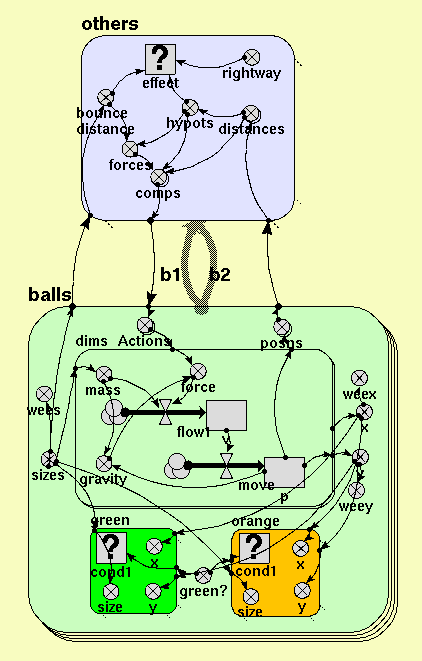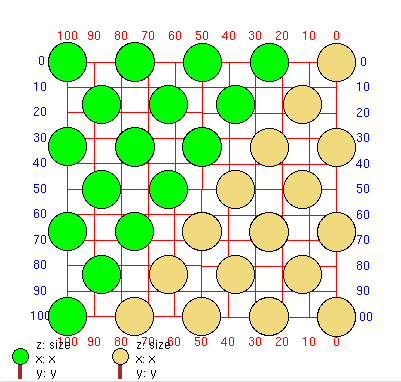# agent-based modelling

## Individual particle based diffusion model

ModelId:
diffusion
SimileVersion:
diffusion

This models molecules in a lattice. They are held in their lattice positions by mutual repulsion. It starts with a low vibrational energy which gradually increases. They are divided into two types for display purposes to illustrate diffusion. To start with they vibrate around their intital positions, then occasionally exchange positions until above a certain energy the fixed positions are lost and they all get mixed.Equations:

Contained submodel(s)
others
balls

Submodel others

Submodel others is a relation submodel for a relation between balls and itself.

Condition for existence of submodel

effect

Units: boolean
effect = hypots<bounce_distance and rightway
Where:
bounce_distance is the variable bounce distance in this submodel.
hypots is the variable hypots in this submodel.
rightway is the variable rightway in this submodel.

Variable(s)

comps

Units: array(1,2)
comps = forces*[distances]/hypots
Where:
forces is the variable forces in this submodel.
hypots is the variable hypots in this submodel.
[distances] is the variable distances in this submodel.

bounce distance

Units: 1
bounce distance = sizes_b1+sizes_b2
Where:
sizes_b1 is the variable sizes in balls
sizes_b2 is the variable sizes in balls

distances

Units: array(1,2)
distances = [posns_b1]-[posns_b2]
Where:
[posns_b1] is the variable posns in balls
[posns_b2] is the variable posns in balls

hypots

Units: 1
hypots = hypot(element([distances],1),element([distances],2))
Where:
[distances] is the variable distances in this submodel.

forces

Units: 1
forces = pow(bounce_distance/hypots,4)
Where:
bounce_distance is the variable bounce distance in this submodel.
hypots is the variable hypots in this submodel.

rightway

Units: boolean
rightway = index(1)<index(2)

Submodel balls

Submodel balls is a fixed membership submodel with 32 members.

Variable(s)

Actions

Units: array(1,2)
Actions = sum({[comps_b1]})-sum({[comps_b2]})
Where:
{[comps_b1]} is the variable comps in others
{[comps_b2]} is the variable comps in others

green?

any(index(1)==[2,3,4,5,7,8,9])
Units: boolean
green? = any(index(1)==[2,3,4,5,7,8,9,12,13,14,17,18,22,23,27,32])

posns

Units: array(1,2)
posns = [p]
Where:
[p] is the compartment p in balls/dims

x

Units: 1
x = element([p],1)
Where:
[p] is the compartment p in balls/dims

y

Units: 1
y = element([p],2)
Where:
[p] is the compartment p in balls/dims

sizes

Units: 1
sizes = 15

wees

Units: 1
wees = sizes/10
Where:
sizes is the variable sizes in this submodel.

weex

Units: 1
weex = x/10+45
Where:
x is the variable x in this submodel.

weey

Units: 1
weey = y/10+45
Where:
y is the variable y in this submodel.

Contained submodel(s)
green
dims
orange

Submodel balls/green

Submodel green is a conditional submodel.

Condition for existence of submodel

cond1

Units: boolean
cond1 = green_
Where:
green_ is the variable green? in balls

Variable(s)

size

Units: 1
size = sizes
Where:
sizes is the variable sizes in balls

x

Units: 1
x = x
Where:
x is the variable x in balls

y

Units: 1
y = y
Where:
y is the variable y in balls

Submodel balls/dims

Submodel dims is a fixed membership submodel with 2 members.

Flow(s)

move
Units: 1
move = v
Where:
v is the compartment
v in this submodel.

flow1
Units: 1
flow1 = force/mass
Where:
force is the variable
force in this submodel.
mass is the variable mass in this submodel.

Variable(s)

gravity

Units: 1
gravity = 10*mass*if p<0 then 0-p elseif p>100 then 100-p else 0
Where:
mass is the variable mass in this submodel.
p is the compartment p in this submodel.

force

Units: 1
force = a+element([Actions],index(1))+rand_var(-10,10)

mass

Units: 1
mass = sizes*sizes/100
Where:
sizes is the variable sizes in balls

Submodel balls/orange

Submodel orange is a conditional submodel.

Condition for existence of submodel

cond1

Units:
cond1 = missing

Variable(s)

size

Units: 1
size = sizes
Where:
sizes is the variable sizes in balls

x

Units: 1
x = x
Where:
x is the variable x in balls

y

Units: 1
y = y
Where:

Results:

These images show the positions of particles at the start and end of the run.